• +91 9971497814
• info@interviewmaterial.com

# Chapter 3- Data Representation Interview Questions Answers

### Related Subjects

Question 1 :

Octal (base 8) was previously a popular choice for representing digital circuit numbers in a form that is more compact than binary. Octal is sometimes abbreviated as oct. Octal counting goes as :
0,1, 2, 3,4, 5, 6, 7,10,11,12,13,14,15,16,17,20, 21 and so on.
Hexadecimal (base 16) is currently the most popular choice for representing digital circuit numbers in a form that is more compact than binary. Hexadecimal numbers are sometimes represented by preceding the value with ‘Ox’, as in 0x1 B84. Hexadecimal is sometimes abbreviated as hex. Hexadecimal counting goes :
0,1,2, 3,4,5, 6, 7, 8,9, A, B, C, D, E, F, and so on.

Question 2 :
Explain decimal and binary number.

Decimal (base 10) is the way most human beings represent numbers. Decimal is sometimes abbreviated as dec. Decimal counting goes :
0,1,2,3,4,5,6,7,8,9,10,11,12,13,14,15,16,17,18 and so on.
Binary (base 2) is the natural way most digital circuits represent and manipulate numbers. Binary numbers are sometimes represented by preceding the value with ‘Ob’, as in Ob1O11. Binary is sometimes abbreviated as bin.
Binary counting goes as : 0,1,10,11,100,101,110,
111, 1000, 1oo1, 1010,1011, 11oo, 1101, 1110, 1111,10000,10001 and so on.

Question 3 :
Convert (39286)10 to octal form.

Answer 3 :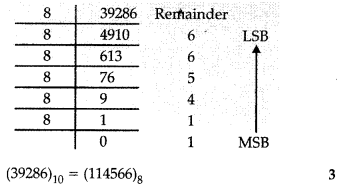(39286)10 = (114566)8

Question 4 :
Convert (0.2)10 to the binary form.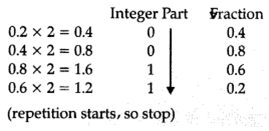(0.2)_10= (0.0011)_2

_

Question 5 :
Convert (423.03125)10 to octal form.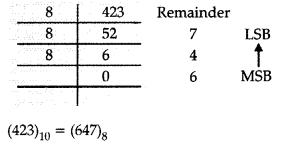second we convert the fraction part by multiplying fraction part by 8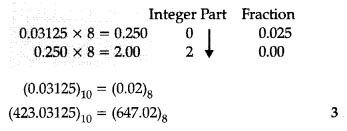Question 6 :

Fill the correctentry.

 Decimal Binary Octal 0 000 0 1 001 1 2 ? 2 3 Oil 3 4 100 4 5 101 5 ? 110 6 7 ? ?

 Decimal Binary Octal 0 000 0 1 001 1 2 010 2 3 Oil 3 4 100 4 5 101 5 6 110 6 7 111 7

Question 7 :
Do as directed :

(a) Convert the Decimal number 781 to its Binary equivalent.
(b) Convert Binary number 101101.001 to its decimal equivalent.
(c) Convert Octal number 321.7 into its Binary equivalent.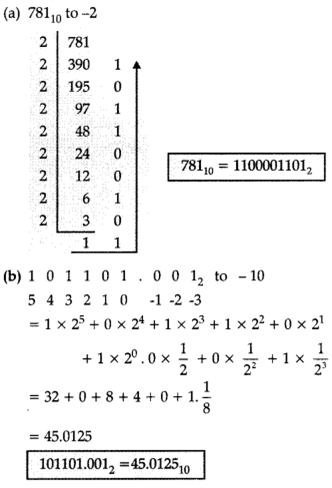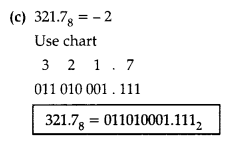Question 8 :
Do as directed :

(a) Covert the Hexadecimal number 3BC into its Binary equivalent
(b) Convert the Binary number 10011010.010101 to its Hexadecimal equivalent.
(c) Convert the Decimal number 345 into Octal number.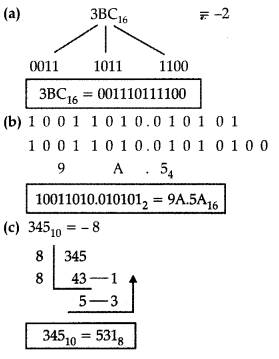Question 9 :
What is ASCII ?

Answer 9 : It is acronym for the American Standard Code for Information Interchange. It is used in most microcomputers and minicomputers and in many mainframes. It is a 7-bit code so it has 27 = 128 possible code groups.

Question 10 :
What is ISCII ?

Answer 10 : It is acronym for Indian Standard Code for Information Interchange. It is a 8-bit code so it has 28 = 256 possible code groups. It retains all ASCII characters and offers codng for Indian characters also.

Todays Deals### Chapter 3- Data Representation Contributorskrishan

Name:
Email:

# Latest News# 9000 interview questions in different categories